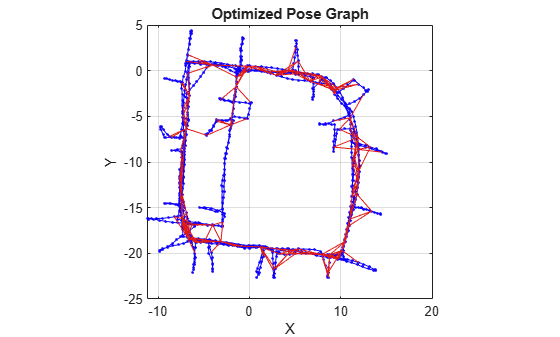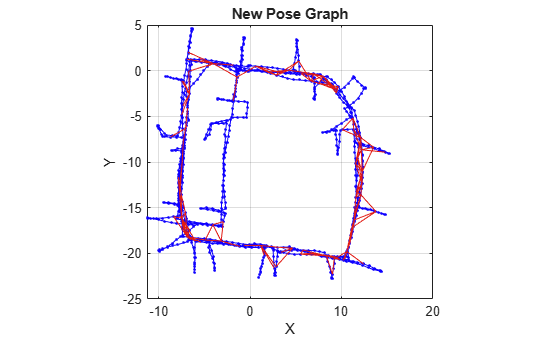Add relative pose to pose graph

Since R2019b

## Syntax

``addRelativePose(poseGraph,measurement)``
``addRelativePose(poseGraph,measurement,infoMat)``
``addRelativePose(poseGraph,measurement,infoMat,fromNodeID)``
``addRelativePose(poseGraph,measurement,infoMat,fromNodeID,toNodeID)``
``[nodePair,edgeID] = addRelativePose(___)``

## Description

````addRelativePose(poseGraph,measurement)` creates a node based on the input `measurement` that connects to the last pose node in the pose graph. To add landmark nodes, see the `addPointLandmark` function.```
``` `addRelativePose(poseGraph,measurement,infoMat)` also specifies the information matrix as part of the edge constraint, which represents the uncertainty of the pose measurement.```
``` `addRelativePose(poseGraph,measurement,infoMat,fromNodeID)` creates a new pose node and connects it to the specific node specified by `fromNodeID`.```

example

``` `addRelativePose(poseGraph,measurement,infoMat,fromNodeID,toNodeID)` creates an edge by specifying a relative pose measurement between existing nodes specified by `fromNodeID` and `toNodeID`. This edge is called a loop closure. If a loop closure already exists, the function appends the new measurement. Calling the `optimizePoseGraph` function combines multiple appended measurements into a single edge. This syntax does not support adding edges to a landmark node.```
``` `[nodePair,edgeID] = addRelativePose(___)` returns the newly added edge and edge ID using any of the previous syntaxes.```

## Examples

collapse all

This example shows how to identify and remove spurious loop closures from pose graph. To do this, you can modify the relative pose of a loop closure edge and try optimizing the pose graph with and without removing the auto spurious loop closure and compare the results.

Load the Intel Research Lab Dataset that contains a 2-D pose graph. Optimize the pose graph. Plot the pose graph with IDs off. Red lines indicate loop closures identified in the dataset.

```load intel-2d-posegraph.mat pg optimizedPG = optimizePoseGraph(pg); show(optimizedPG,IDs="off"); title("Optimized Pose Graph")```Modify the relative pose of the loop closure edge `1386` to some random values.

```loopclosureId = 1386; nodePair = edgeNodePairs(optimizedPG,loopclosureId); [relPose,infoMat] = edgeConstraints(optimizedPG,loopclosureId); relPose(2) = -5; relPose(3) = 1.5; addRelativePose(optimizedPG,relPose,infoMat,nodePair(1),nodePair(2));```

Optimize the pose graph without auto loop closure trimming. Plot the optimized pose graph to see the poor adjustment of the nodes with loop closures.

```[updatedPG,solutionInfo] = optimizePoseGraph(optimizedPG); show(updatedPG,IDs="off"); title("Updated Pose Graph")```Certain loop closures should be trimmed from the pose graph. Use the `trimLoopClosures` function to trim these bad loop closures. Set the truncation threshold and maximum iterations for the trimmer parameters.

`trimParams = struct("TruncationThreshold",0.5,"MaxIterations",100);`

Generate solver options.

`solverOptions = poseGraphSolverOptions("g2o-levenberg-marquardt");`

Use the `trimLoopClosures` function with the trimmer parameters and solver options. Plot the new pose graph to see the bad loop closures were removed.

```[newPG,trimInfo] = trimLoopClosures(updatedPG,trimParams,solverOptions); show(newPG,IDs="off"); title("New Pose Graph")```## Input Arguments

collapse all

Pose graph, specified as a `poseGraph` or `poseGraph3D` object.

Relative pose between nodes, specified as one of the following:

For `poseGraph` (2-D), the pose is a `[x y theta]` vector, which defines a xy-position and orientation angle, `theta`.

For `poseGraph3D`, the pose is a ```[x y z qw qx qy qz]``` vector, which defines by an xyz-position and quaternion orientation, ```[qw qx qy qz]```

Note

Many other sources for 3-D pose graphs, including `.g2o` formats, specify the quaternion orientation in a different order, for example, `[qx qy qz qw]`. Check the source of your pose graph data before adding nodes to your `poseGraph3D` object.

Information matrices, specified in compact form as a six-element vector or 21-element vector.

Each vector is the compact form of the upper triangle of the square information matrix. An information matrix represents the uncertainty of the measurement. The matrix is calculated as the inverse of the covariance. If the measurement is an `[x y theta]` vector, the covariance matrix is a 3-by-3 of pairwise covariance calculations. Typically, the uncertainty is determined by the sensor model.

For `poseGraph` (2-D), each information matrix is a six-element vector. The default is `[1 0 0 1 0 1]`. For landmark nodes, the last three elements are returned as `NaN`.

For `poseGraph3D`, each information matrix is a 21-element vector. The default is ```[1 0 0 0 0 0 1 0 0 0 0 1 0 0 0 1 0 0 1 0 1]```.

Node to attach from, specified as a positive integer. This integer corresponds to the node ID of a node in `poseGraph`. When specified without `toNodeID`, `addRelativePose` creates a new node and adds an edge between the new node and the `fromNodeID` node.

Node to attach to, specified as a positive integer. This integer corresponds to the node ID of a node in `poseGraph`. `addRelativePose` adds an edge between this node and the `fromNodeID` node.

## Output Arguments

collapse all

Edge node pairs in pose graph, returned as two-element vector that lists the IDs of the two nodes that each edge connects. Multiple edges may exist between the same pair of nodes.

ID of added edge, returned as a positive integer.

## Version History

Introduced in R2019b